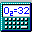##Percent Solver Mode

Note: Percent Solver Mode is disabled if the "Treat Brackets as Parentheses" option is selected in the Program Preferences screen.

The Percent Solver Mode is enabled using the View Menu or using the ShortCut Keys The F11, PAGE UP or PAGE DOWN. Percent solver mode is useful for entering target percent composition values and solving for the best value of "x" in a formula to satisfy these values. The equation for determining the best value for x is found in the calculations section of this help file.

To set the target percent composition values, use the PAGE UP, PAGE DOWN, and arrow keys to move among the percent compositions shown, then press Enter or click on a percent to type in the target values for the solver to aim for. Select OK to accept the value, cancel to cancel any changes, or Reset to un-target the percent.

Before the solver can work, you need to place an x in the formula, directly after a bracket. For example, "C6H6-[xH2O]" is useful for calculating the amount of water in a benzene sample, provided elemental analysis data is available. The formula must be in bracketed form for the solver to work. If you have the formula "NaClx", where you wish to find the number of chlorine atoms based on data, simply retype the formula in the form "Na[xCl]" for the percent solver to be able to interpret the it. When at least one target percent is entered and an x is present after a bracket in the formula, you may navigate the cursor to the formula line then press ENTER or click on the Calculate Button to begin solving.

The program will indicate the progress of its calculations. Press any key or click the mouse during the calculations to stop them before they are completed. The computer should stop calculating once it gets the same answer four times in a row, which should be to approximately four or five decimal places.

After the calculations are complete, a window will appear which shows the obtained percentages, target percentages, and differences between these numbers. This is useful for seeing how well the x value works for matching the target percentages. Use the Copy button to copy the data to the clipboard. Closing the box with the close button returns you to the main program. Press the F11 key again to exit percent solver mode.

NOTE: If the program is not in Percent Solver mode, but an x is present in the formula after a bracket, the program will calculate the molecular weight based on x equaling one.